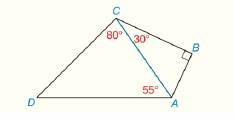Chapter 3.CR, Problem 18CR### Elementary Geometry for College St...

6th Edition
Daniel C. Alexander + 1 other
ISBN: 9781285195698

#### Solutions

Chapter
Section### Elementary Geometry for College St...

6th Edition
Daniel C. Alexander + 1 other
ISBN: 9781285195698
Textbook Problem
1 views

# Name the longest line segment shown in quadrilateral A B C D .To determine

The longest line segment shown in quadrilateral ABCD.

Explanation

Procedure used:

The sum of interior angles of the triangle is 180°.

If the measure of one angle of a triangle is greater than the measure of a second angle, than the side opposite to the larger angle is longer than the side opposite to the smaller angle.

Given:

The quadrilateral ABCD is shown in the figure below,

Figure (1)

Calculation:

From the given figure (1),

Consider the triangle ΔACD.

Write the equation to calculate the sum of angles of the triangle.

DAC+ACD+D=180° (1)

Substitute 80° for ACD and 55° for DAC in equation (1) to calculate the sum of angles of the triangle.

55°+80°+D=180°D=180°135°=45°

Since, the side opposite to the larger angle is longer and the side opposite to the smaller angle is smaller. Then,

The side DA¯ is longer and the side AC¯ is smaller

### Still sussing out bartleby?

Check out a sample textbook solution.

See a sample solution

#### The Solution to Your Study Problems

Bartleby provides explanations to thousands of textbook problems written by our experts, many with advanced degrees!

Get Started

#### Evaluate the expression sin Exercises 116. 323

Finite Mathematics and Applied Calculus (MindTap Course List)

#### 2 1 0 does not exist

Study Guide for Stewart's Multivariable Calculus, 8th

#### By determining the limit of Riemann sums, the exact value of dx is a) 7 b) 9 c) d) 18

Study Guide for Stewart's Single Variable Calculus: Early Transcendentals, 8th

#### What is the goal of a single-case experimental research design?

Research Methods for the Behavioral Sciences (MindTap Course List)